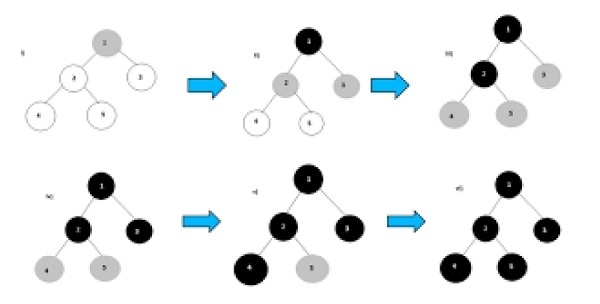# BFS using STL for competitive coding in C++?

C++Server Side ProgrammingProgramming

The Breadth First Search (BFS) traversal is an algorithm, which is used to visit all of the nodes of a given graph. In this traversal algorithm one node is selected and then all of the adjacent nodes are visited one by one. After completing all of the adjacent vertices, it moves further to check another vertices and checks its adjacent vertices again.In The competitive coding, we have to solve problems very quickly. We will use the STL (Standard Library of C++) to implement this algorithm, we need to use the Queue data structure. All the adjacent vertices are added into the queue, when all adjacent vertices are completed, one item is removed from the queue and start traversing through that vertex again.

In Graph sometimes, we may get some cycles, so we will use an array to mark when a node is visited already or not.

Input : The Adjacency matrix of the graph.
A B C D E F
A 0 1 1 1 0 0
B 1 0 0 1 1 0
C 1 0 0 1 0 1
D 1 1 1 0 1 1
E 0 1 0 1 0 1
F 0 0 1 1 1 0
Output : BFS Traversal: B A D E C F

## Algorithm

### bfs(vertices, start)

Input − The list of vertices, and the start vertex.

Output − Traverse all of the nodes, if the graph is connected.

Begin
define an empty queue que
at first mark all nodes status as unvisited
add the start vertex into the que
while que is not empty, do
delete item from que and set to u
display the vertex u
for all vertices 1 adjacent with u, do
if vertices[i] is unvisited, then
mark vertices[i] as temporarily visited
mark
done
mark u as completely visited
done
End

## Example

Live Demo

#include<iostream>
#include<queue>
#define NODE 6
using namespace std;
class node {
public:
int val;
int state; //status
};
int graph[NODE][NODE] = {
{0, 1, 1, 1, 0, 0},
{1, 0, 0, 1, 1, 0},
{1, 0, 0, 1, 0, 1},
{1, 1, 1, 0, 1, 1},
{0, 1, 0, 1, 0, 1},
{0, 0, 1, 1, 1, 0}
};
void bfs(node *vert, node s) {
node u;
int i, j;
queue<node> que;
for(i = 0; i<NODE; i++) {
vert[i].state = 0; //not visited
}
vert[s.val].state = 1;//visited
que.push(s); //insert starting node
while(!que.empty()) {
u = que.front(); //delete from queue and print
que.pop();
cout << char(u.val+'A') << " ";
for(i = 0; i<NODE; i++) {
if(graph[i][u.val]) {
//when the node is non-visited
if(vert[i].state == 0) {
vert[i].state = 1;
que.push(vert[i]);
}
}
}
u.state = 2;//completed for node u
}
}
int main() {
node vertices[NODE];
node start;
char s;
for(int i = 0; i<NODE; i++) {
vertices[i].val = i;
}
s = 'B';//starting vertex B
start.val = s-'A';
cout << "BFS Traversal: ";
bfs(vertices, start);
cout << endl;
}

## Output

BFS Traversal: B A D E C F